# IB DP Physics: SL复习笔记4.1.1 Properties of Oscillations

### Describing Oscillations

• An oscillation is defined as follows:

The repetitive variation with time t of the displacement x of an object about the equilibrium position (x = 0)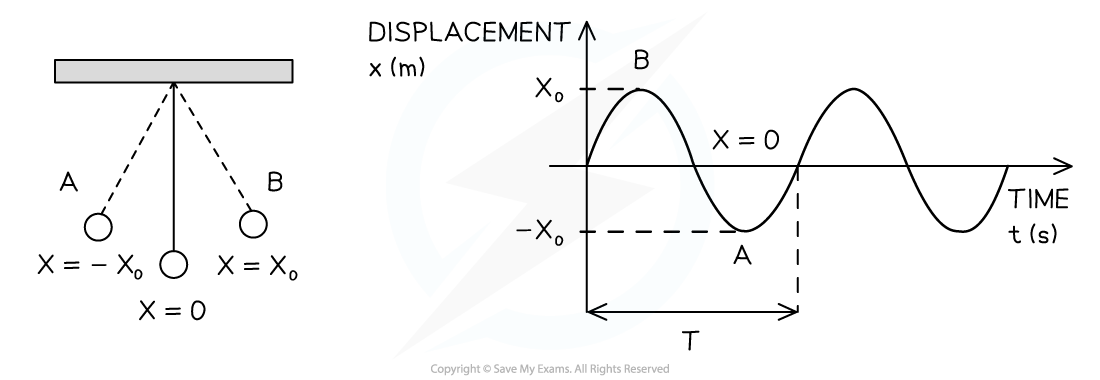A pendulum oscillates between A and B. On a displacement-time graph, the oscillating motion of the pendulum is represented by a wave, with an amplitude equal to x0

• Displacement (x) of a wave is the distance of a point on the wave from its equilibrium position
• It is a vector quantity; it can be positive or negative and it is measured in metres (m)
• Period (T) or time period, is the time interval for one complete repetition and it is measured in seconds (s)
• If the oscillations have a constant period, they are said to be isochronous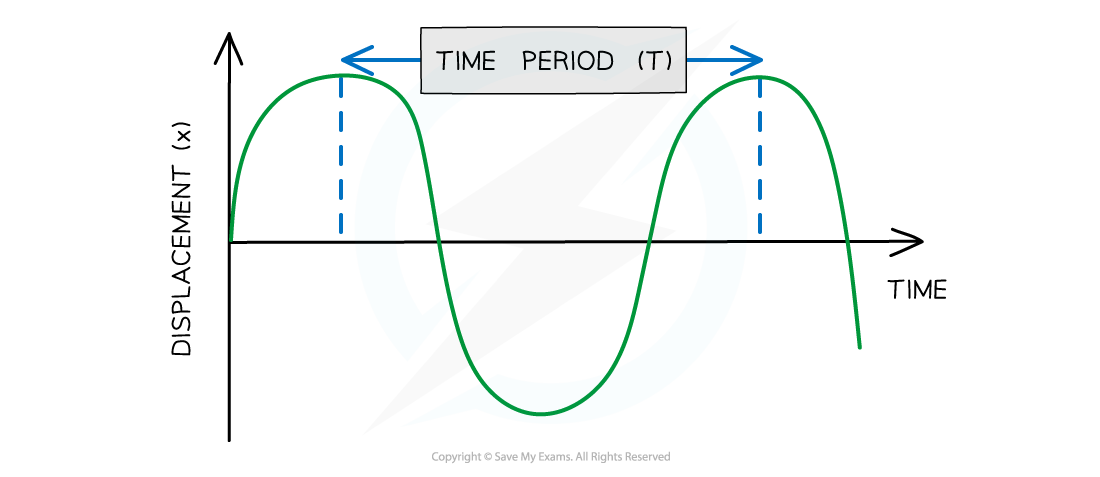Diagram showing the time period of a wave

• Amplitude (x0) is the maximum value of the displacement on either side of the equilibrium position is known as the amplitude of the oscillation
• Amplitude is measured in metres (m)
• Wavelength (λ) is the length of one complete oscillation measured from the same point on two consecutive waves
• Wavelength is measured in metres (m)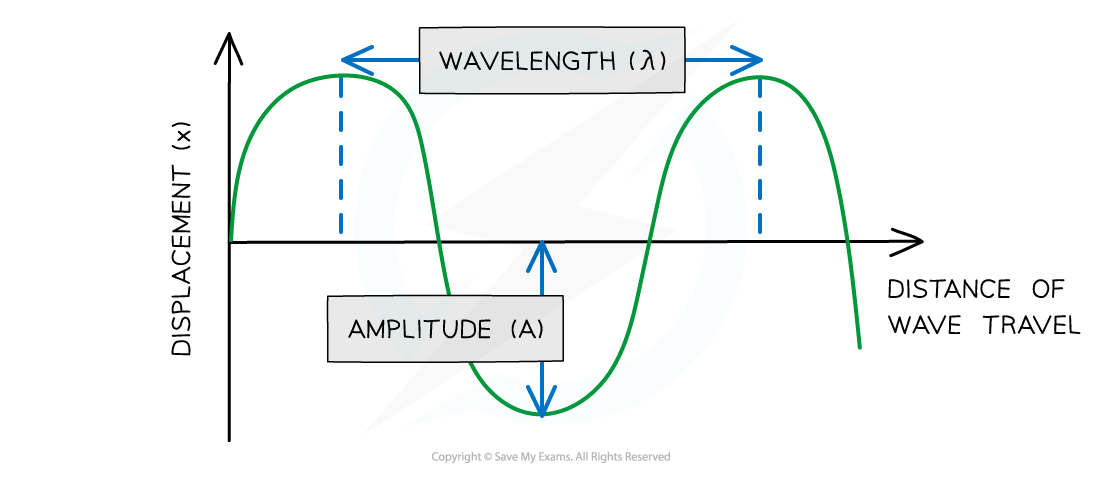• Frequency (f) is the number of oscillations per second and it is measured in hertz (Hz)
• Hz have the SI units of per second s−1
• The frequency and the period of the oscillations are related by the following equation:#### Phase & Phase Difference

• Phase is a useful way to think about waves
• The phase of a wave can be considered in terms of:
• Wavelength
• Degrees
• One complete oscillation is:
• 1 wavelength
• 360°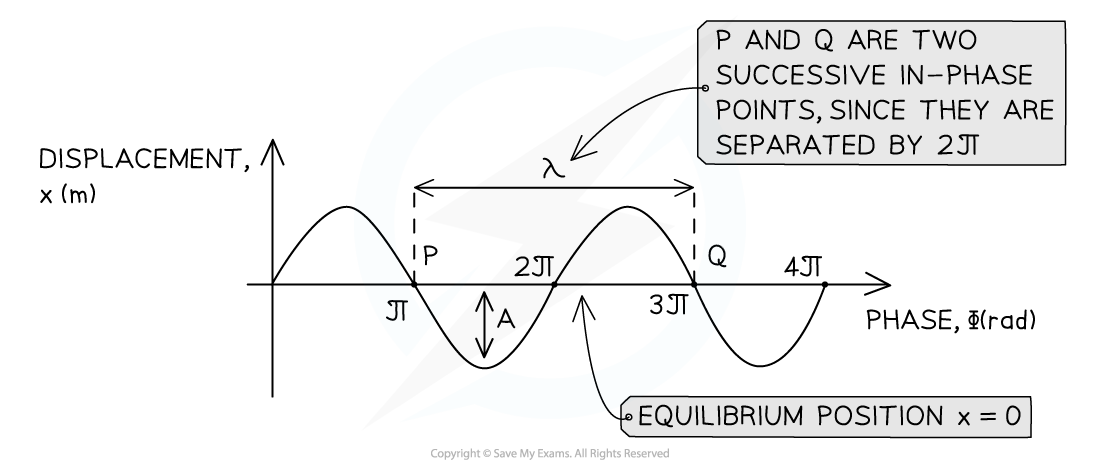Wavelength λ and amplitude A of a travelling wave

• The phase difference between two waves is a measure of how much a point or a wave is in front or behind another
• This can be found from the relative position of the crests or troughs of two different waves of the same frequency
• When the crests of each wave, or the troughs of each wave are aligned, the waves are in phase
• When the crest of one wave aligns with the trough of another, they are in antiphase
• The diagram below shows the green wave leads the purple wave by ¼ λ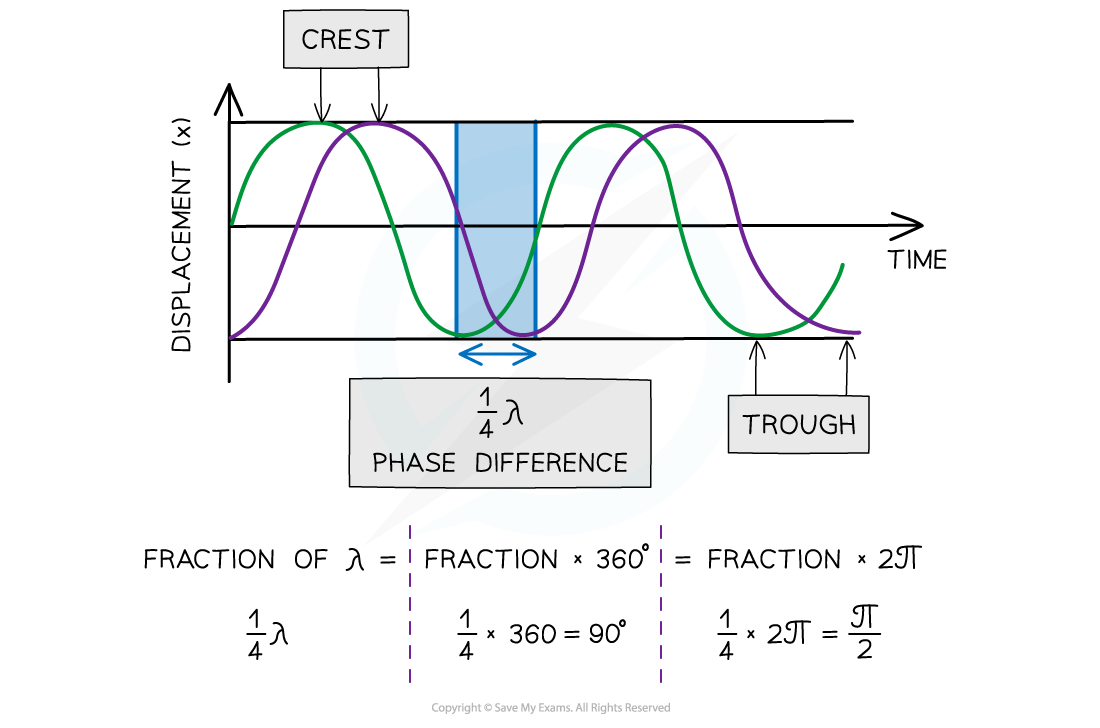Two waves ¼ λ out of phase

• In contrast, the purple wave is said to lag behind the green wave by ¼ λ
• Phase difference is measured in fractions of a wavelength, degrees or radians
• The phase difference can be calculated from two different points on the same wave or the same point on two different waves
• The phase difference between two points can be described as:
• In phase is 360o or 2π radians
• In anti-phase is 180o or π radians

#### Worked Example

A child on a swing performs 0.2 oscillations per second.Calculate the period of the child's oscillations.

Step 1: Write down the frequency of the child's oscillations

f = 0.2 Hz

Step 2: Write down the relationship between the period T and the frequency f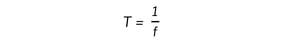Step 3: Substitute the value of the frequency into the above equation and calculate the period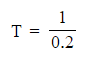T = 5 s

#### Worked Example

Plane waves on the surface of water at a particular instant are represented by the diagram below.The waves have a frequency of 2.5 Hz.Determine:

a) The amplitude

b) The wavelength

c) The phase difference between points A and B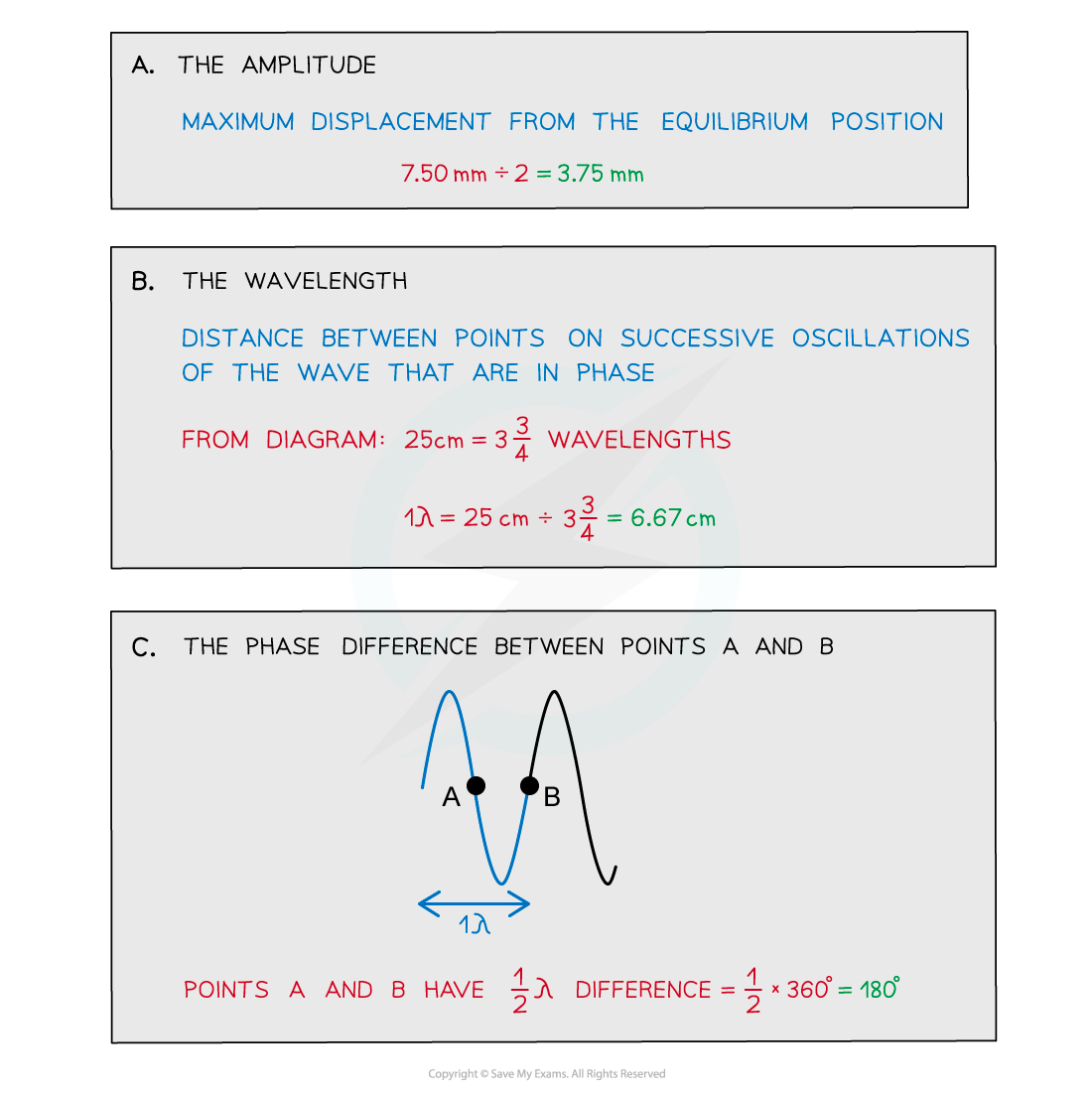#### Exam Tip

When labelling the wavelength and time period on a diagram:

• Make sure that your arrows go from the very top of a wave to the very top of the next one
• If your arrow is too short, you will lose marks
• The same goes for labelling amplitude, don’t draw an arrow from the bottom to the top of the wave, this will lose you marks too.